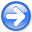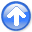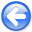Fast log-decon with a quasi-Newton solver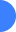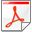Next: Two solvers Up: Guitton: Quasi-Newton log-decon Previous: Guitton: Quasi-Newton log-decon

# INTRODUCTION

The log-decon method of Claerbout et al. (2011) estimates a filter that can both handle non-minimum phase wavelets (e.g., Ricker) and produce sparse seismic reflections where the polarity is easily identifiable. This method is extended in Claerbout et al. (2012) to include variable gain. Claerbout proposes to compute the filter coefficients with a steepest-descent approach, where the step length can be estimated very accurately with a Newton-search technique. Steepest descent is notoriously slow: its convergence rate depends on the conditioning of the problem producing a well-known zig-zagging effect close to the solution. Here, I propose to employ the L-BFGS method, a quasi-Newton technique that improves the convergence and decreases the number of iterations.

2012-10-29